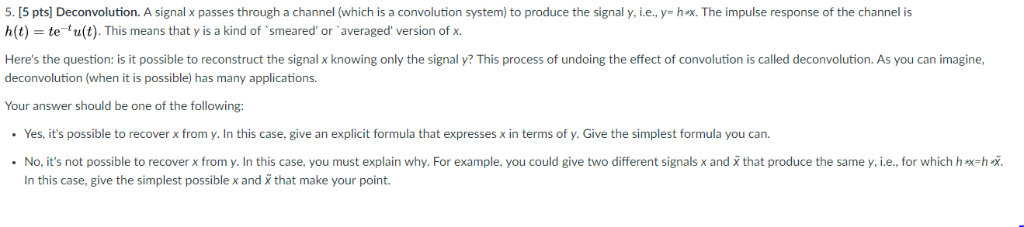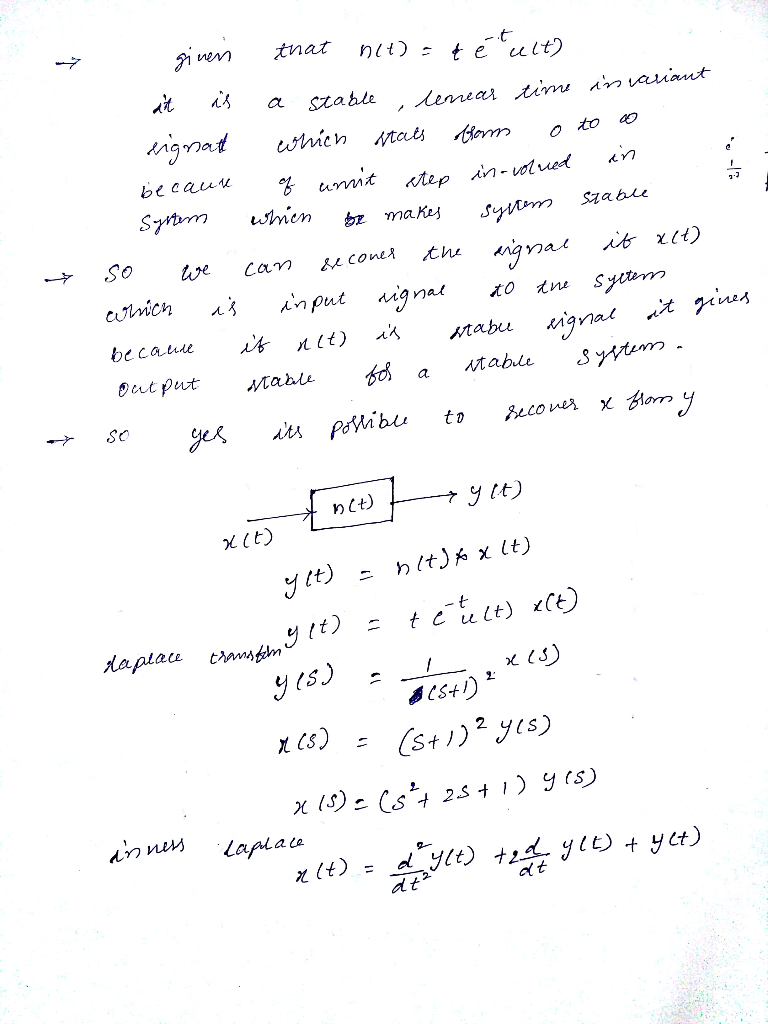# 5. [5 pts] Deconvolution. A signal x passes through a channel (which is a convolution system) to ...5. [5 pts] Deconvolution. A signal x passes through a channel (which is a convolution system) to produce the signal y, i.e., y- hx. The impulse response of the channel is h(t) = te-tu(t). This means that y is a kind of 'smeared' or 'averaged' version of x. Here's the question: is it possible to reconstruct the signal x knowing only the signal y? This process of undoing the effect of convolution is called deconvolution. As you can imagine, deconvolution (when it is possible) has many applications Your answer should be one of the following: . Yes, it's possible to recover x from y. In this case, give an explicit formula that expresses x in terms of y. Give the simplest formula you can. No, it's not possible to recover x from y. In this case, you must explain why. For example, you could give two different signals x andX that produce the same y, i.e., for which h-hx In this case, give the simplest possible x and X that make your point.answer is uploaded above

##### Add Answer of: 5. [5 pts] Deconvolution. A signal x passes through a channel (which is a convolution system) to ...
More Homework Help Questions Additional questions in this topic.

• #### 8 8.6-4 For a DSB-SC system with a channel noise PSD of Sn() 10-12 and a baseband signal of bandw...

Need Online Homework Help?